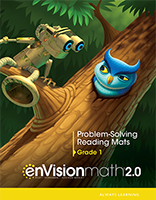1 Topic 1 Solve Addition and Subtraction Problems to 10 Lesson 1-1:  Solve Problems: Add To Also consider: Lesson 1-1:  Solve Problems: Add To Also consider: Lesson 1-2:  Solve Problems: Put Together Also consider: Lesson 1-2:  Solve Problems: Put Together Also consider: Lesson 1-3:  Solve Problems: Both Addends Unknown Lesson 1-3:  Solve Problems: Both Addends Unknown Lesson 1-4:  Solve Problems: Take From Also consider: Lesson 1-4:  Solve Problems: Take From Also consider: Lesson 1-5:  Solve Problems: Compare Situations Also consider: Lesson 1-5:  Solve Problems: Compare Situations Also consider: Lesson 1-6:  Continue to Solve Problems: Compare Situations Lesson 1-6:  Continue to Solve Problems: Compare Situations Lesson 1-7:  Practice Solving Problems: Add To Lesson 1-7:  Practice Solving Problems: Add To Lesson 1-8:  Solve Problems: Put Together/Take Apart Lesson 1-8:  Solve Problems: Put Together/Take Apart Lesson 1-9:  Math Practices & Problem Solving: Construct Arguments Also consider: Lesson 1-9:  Math Practices & Problem Solving: Construct Arguments Also consider:
 2 Topic 2 Develop Fluency: Addition and Subtraction Facts within 10 Lesson 2-1:  Count On to Add Lesson 2-1:  Count On to Add Lesson 2-2:  Doubles Lesson 2-2:  Doubles Lesson 2-3:  Near Doubles Lesson 2-3:  Near Doubles Lesson 2-4:  Facts with 5 on a Ten-Frame Also consider: Lesson 2-4:  Facts with 5 on a Ten-Frame Also consider: Lesson 2-5:  Add in Any Order Lesson 2-5:  Add in Any Order Lesson 2-6:  Count Back to Subtract Lesson 2-6:  Count Back to Subtract Lesson 2-7:  Think Addition to Subtract Also consider: Lesson 2-7:  Think Addition to Subtract Also consider: Lesson 2-8:  Continue to Think Addition to Subtract Lesson 2-8:  Continue to Think Addition to Subtract Lesson 2-9:  Solve Word Problems with Facts to 10 Lesson 2-9:  Solve Word Problems with Facts to 10 Lesson 2-10:  Math Practices & Problem Solving: Look For & Use Structure Also consider: Lesson 2-10:  Math Practices & Problem Solving: Look For & Use Structure Also consider:
 4 Topic 4 Subtraction Facts to 20: Use Strategies Lesson 4-1:  Count to Subtract Lesson 4-1:  Count to Subtract Lesson 4-2:  Make 10 to Subtract Lesson 4-2:  Make 10 to Subtract Lesson 4-3:  Continue to Make 10 to Subtract Lesson 4-3:  Continue to Make 10 to Subtract Lesson 4-4:  Fact Families Also consider: Lesson 4-4:  Fact Families Also consider: Lesson 4-5:  Use Addition to Subtract Lesson 4-5:  Use Addition to Subtract Lesson 4-6:  Continue to Use Addition to Subtract Lesson 4-6:  Continue to Use Addition to Subtract Lesson 4-7:  Explain Subtraction Strategies Also consider: Lesson 4-7:  Explain Subtraction Strategies Also consider: Lesson 4-8:  Solve Word Problems with Facts to 20 Lesson 4-8:  Solve Word Problems with Facts to 20 Lesson 4-9:  Math Practices & Problem Solving: Reasoning Also consider: Lesson 4-9:  Math Practices & Problem Solving: Reasoning Also consider:
 5 Topic 5 Work with Addition and Subtraction Equations Lesson 5-1:  Find the Unknown Numbers Also consider: Lesson 5-1:  Find the Unknown Numbers Also consider: Lesson 5-2:  True or False Equations Also consider: Lesson 5-2:  True or False Equations Also consider: Lesson 5-3:  Make True Equations Lesson 5-3:  Make True Equations Lesson 5-4:  Word Problems with Three Addends Lesson 5-4:  Word Problems with Three Addends Lesson 5-5:  Add Three Numbers Lesson 5-5:  Add Three Numbers Lesson 5-6:  Solve Addition and Subtraction Word Problems Lesson 5-6:  Solve Addition and Subtraction Word Problems Lesson 5-7:  Math Practices & Problem Solving: Precision Lesson 5-7:  Math Practices & Problem Solving: Precision
 6 Topic 6 Represent and Interpret Data Lesson 6-1:  Organize Data into Three Categories Lesson 6-1:  Organize Data into Three Categories Lesson 6-2:  Collect and Represent Data Lesson 6-2:  Collect and Represent Data Lesson 6-3:  Interpret Data Lesson 6-3:  Interpret Data Lesson 6-4:  Continue to Interpret Data Lesson 6-4:  Continue to Interpret Data Lesson 6-5:  Math Practices & Problem Solving: Persevere Lesson 6-5:  Math Practices & Problem Solving: Persevere
 7 Topic 7 Extend the Counting Sequence Lesson 7-1:  Count by 10s to 120 Also consider: Lesson 7-1:  Count by 10s to 120 Also consider: Lesson 7-2:  Count by 1s to 120 Also consider: Lesson 7-2:  Count by 1s to 120 Also consider: Lesson 7-3:  Count on a Number Chart to 120 Lesson 7-3:  Count on a Number Chart to 120 Lesson 7-4:  Count by 1s or 10s to 120 Lesson 7-4:  Count by 1s or 10s to 120 Lesson 7-5:  Count on an Open Number Line Also consider: Lesson 7-5:  Count on an Open Number Line Also consider: Lesson 7-6:  Count and Write Numerals Lesson 7-6:  Count and Write Numerals Lesson 7-7:  Math Practices & Problem Solving: Repeated Reasoning Lesson 7-7:  Math Practices & Problem Solving: Repeated Reasoning
 8 Topic 8 Understand Place Value Lesson 8-1:  Make Numbers 11 to 19 Also consider: Lesson 8-1:  Make Numbers 11 to 19 Also consider: Lesson 8-2:  Numbers Made with Tens Lesson 8-2:  Numbers Made with Tens Lesson 8-3:  Count with Groups of Tens and Leftovers Lesson 8-3:  Count with Groups of Tens and Leftovers Lesson 8-4:  Tens and Ones Also consider: Lesson 8-4:  Tens and Ones Also consider: Lesson 8-5:  Continue with Tens and Leftovers Also consider: Lesson 8-5:  Continue with Tens and Leftovers Also consider: Lesson 8-6:  Math Practices & Problem Solving: Look For & Use Structure Lesson 8-6:  Math Practices & Problem Solving: Look For & Use Structure
 9 Topic 9 Compare Two-Digit Numbers Lesson 9-1:  1 More, 1 Less; 10 More, 10 Less Lesson 9-1:  1 More, 1 Less; 10 More, 10 Less Lesson 9-2:  Make Numbers on a Hundred Chart Lesson 9-2:  Make Numbers on a Hundred Chart Lesson 9-3:  Compare Numbers Lesson 9-3:  Compare Numbers Lesson 9-4:  Compare Numbers with Symbols (>, <, =) Lesson 9-4:  Compare Numbers with Symbols (>, <, =) Lesson 9-5:  Compare Numbers on a Number Line Also consider: Lesson 9-5:  Compare Numbers on a Number Line Also consider: Lesson 9-6:  Math Practices & Problems Solving: Make Sense & Persevere Lesson 9-6:  Math Practices & Problems Solving: Make Sense & Persevere